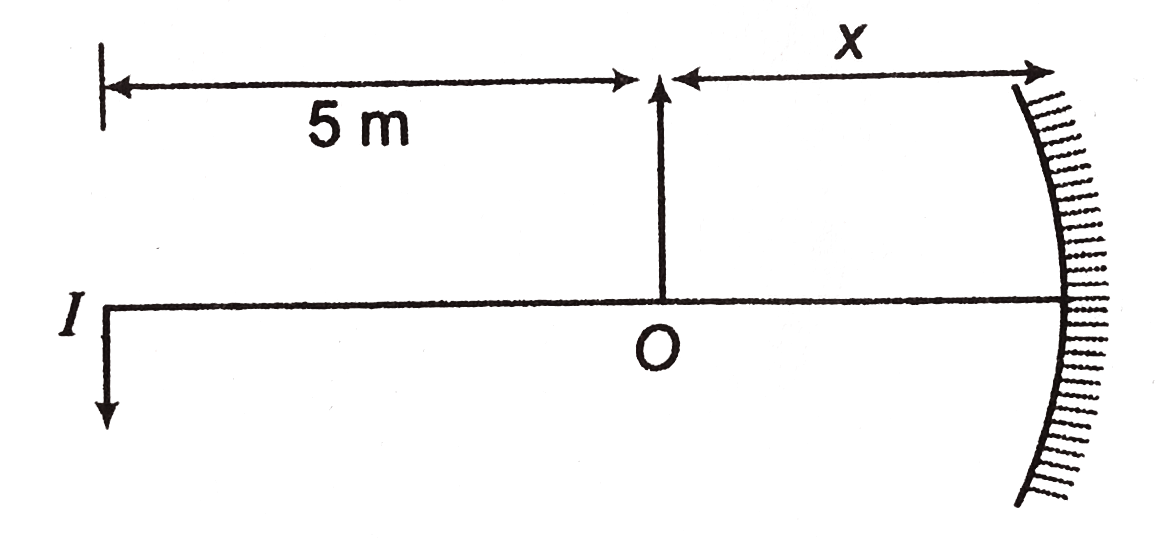# A spherical mirror is used to obtain an image on a screen. The height of the image is 5 times that of the object. What is the type of mirror and at what distance is the object placed from the mirror?

Dear student,

a)
Here in this case the mirror is a concave mirror as image is magnnified.

(b)Image is 5 times magnified.
Hence, v=5u
or, (5+x)=5x
Solving we get,
x=1.25cm
Now using, 1/v+1/u=1/f=2R

### 1/f=1/1+ (1/5) = (6/5)

Or   f= (5.0/6) =0.83m

Regards.

• -1
What are you looking for?